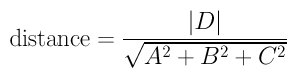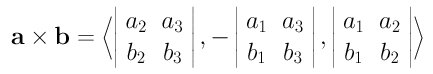Coordinate Systems, Points, Lines and Planes

# Coordinate Systems, Points, Lines and Planes## Two-Dimensional Objects

### Points

The xy-coordinate plane has two coordinate axes, the x- and y-axis. They are perpendicular to each other. Non-perpendicular axes can be used; but, the computation cost is higher.

A point in the xy-plane is represented by two numbers, (x, y), where x and y are the coordinates of the x- and y-axes.

### Lines

A line in the xy-plane has an equation as follows:
Ax + By + C = 0
It consists of three coefficients A, B and C. C is referred to as the constant term. If B is non-zero, the line equation can be rewritten as follows:
y = m x + b
where m = -A/B and b = -C/B. This is the well-known slope-intercept form in which m and b are the slope and the intercept (i.e., the intersection point of the line and the y-axis). If B is zero, the line equation becomes Ax + C = 0, which is a line parallel to the y-axis and intersects the x-axis at point (0,-C/A).

The line equation has three coefficients; but, there are only two independent ones. That is, given a line equation, dividing the equation by one of its non-zero coefficients will not change the line. For example, the line equation 4x+5y+7=0 is equivalent to x+1.25y+1.75=0 and 0.8x+y+1.4=0. Dividing an equation by a non-zero constant is usually referred to as normalization. There is one normalization that is very important to us. It is used to compute the distance between the origin to a line.

Suppose the line equation is Ax+By+C=0, the distance between the origin and the line is given as follows:Thus, after normalizing the line equation by dividing it with the square root of the sum of the squares of A and B, the absolute value of the new constant term is the distance between the origin and the given line.

### Parallel and Perpendicular Lines

Given two lines as follows:
Ax + By + C = 0
Ex + Fy + G = 0
they are parallel to each other if their slopes are equal. Since the slopes are -A/B and -E/F (if B and F are both non-zero), two lines are parallel to each other if and only if the following holds
AF=BE
Note that we can assume that B or F are both non-zero. Otherwise, if both are zero, the lines are parallel to the y-axis and hence are parallel to each other.

Two lines are perpendicular if and only if the product of their slopes is -1. With the line equations above, two lines are perpendicular to each other if and only if the following holds:

AE=-BF## Three-Dimensional Objects

### Points

The coordinate system in space needs three coordinate axes, the x-, y- and z-axis. Therefore, a point in space has three components (x,y,z), where x, y and z are the coordinates of the x-, y- and z-axis.

### Planes

A plane in space has an equation as follows:
Ax + By + Cz + D = 0
It consists of four coefficients A, B, C and D, where D is the constant term. Similar to the line case, the distance between the origin and the plane is given asThe normal vector of a plane is its gradient. The gradient of an equation f(x,y,z)=0 is defined as follows:For a plane, its normal vector is simply <A, B, C>.

Unfortunately, a line in space cannot be represented with a single equation. However, it can be considered as the intersection of two planes. To ease our discussion, we shall switch to using vectors, although this traditional notation is still very useful.## Vectors

Vectors have a very important advantage in geometric computing, because it is "coordinate free." The meaning of "coordinate free" will be clear in later discussions. All vectors will be in boldface like a and A.

A vector is similar to a point. If it is a vector in the plane (resp., space), it has two (resp., three) components. Thus, a vector in a n-dimensional space has n components. For our applications, we shall distinguish two types of vectors: position vectors and direction vectors. A position vector gives the position of a point. More precisely, a point is a vector. A direction vector gives a direction. Hence, it is not a point. In what follows, position vectors and direction vector are written with boldface upper case and lower case, respectively. For example, A and a are position and direction vectors, respectively. In many cases, such distinction is unnecessary.

As you have learned in linear algebra or in calculus, you can add and subtract vectors; but you can only multiply or divide a vector with constants.

The length of a vector is the square root of the sum of squares of all components. A unit-length vector is a vector whose length is one. A vector can be normalized by dividing its components with its length, converting the given vector to a unit-length one while keeping its direction the same. For example, if a=<3, 4, 5>, then the length of a, usually written as |a|, is SQRT(50) and the normalized a is <3/SQRT(50), 4/SQRT(50), 5/SQRT(50)>.

### Inner Product

Given two vectors a and b, its inner product, written as a.b, is the sum of the products of corresponding components. For example, if a = <1,2,3> and b = <2,-1,4>, then a.b = 1*2 + 2*(-1) + 3*4 = 12.

The geometric meaning of the inner product of a and b is the following:

a.b = |a|.|b|cos(t)
More precisely, the inner product of a and b is equal to the product of the length of a, the length of b, and the cosine of the angle t between a and b. This is an important formula, because we know the following facts about a and b once the inner product becomes available:
1. If a.b is zero, where a and b are non-zero vectors, then cos(t) must be zero and, as a result, t must be 90 degree. Therefore, a and b are perpendicular to each other.
2. If a.b is equal to the product of lengths of a and b, the cosine of t is 1 and t is 0 degree. As a result, a and b are parallel to each other and point to the same direction.
3. If a.b is equal to the negative product of lengths of a and b, the cosine of t is -1 and a and b are parallel to each other but point to opposite directions.

### Lines

A line is defined by a based point B and a direction vector d which gives the direction of the line. Therefore, the vector equation of a line is
B + td
where t is a parameter. In many applications, the direction vector is of unit-length.

### Planes

A plane, in its vector form, is specified by a based point B and its normal vector n. For an arbitrary point, or position vector, X on the plane, the direction vector from the base point B to X, X-B, must be perpendicular to the normal vector n. Therefore, we have (X-B).n must be zero. From (X-B).n=0, we have the equation of a plane specified with a base point and its normal vector:
X.n - B.n = 0

Given the vector notation of lines and planes, it is very easy to compute the intersection point of a line and a plane. Let the given line be A+td. Let the plane be defined with a base point B and its normal vector n. Then, this plane has equation X.n=B.n. If the line intersects the plane, there must be a value of t such that the corresponding point lies on the plane. That is, there must be a t such that the point corresponding to this t would satisfy the plane equation. Since a point on the line is A+td, plugging A+td into the plane equation yields

(A+td).n - B.n = 0
Rearranging the terms and solving for t yields
t = (B-A).n / d.n
Therefore, plugging this t into the line equation yields the intersection point.

In the above, if d.n is zero, t cannot be solved and consequently no intersection point exists. The meaning of d.n = 0 is that d and n are perpendicular to each other. Since n is the normal vector of a plane and d is perpendicular to n, d must be parallel to the plane. If the line is parallel to the plane, no intersection point exists.

### Cross Product

There is yet one more important concept about vector: the cross product of two vectors. Given two vectors a and b, their cross product, written as a × b, is defined as follows:where a = < a1, a2, a3 >, b = < b1, b2, b3 >, and | | is a 2-by-2 determinant. In other words, the cross product is

a × b = < a2b3 - a3b2, -(a1b3 - a3b1), a1b2 - a2b1 >
The cross product of a and b, a × b, is perpendicular to both a and b and points to the direction based on the right-handed system in the order of a, b, a × b. Therefore, b × a points to the opposite direction of a × b. The length of a × b is |a| |b| sin(t), where t is the acute angle between a and b. Hence, if a and b are perpendicular to each other, the length of a × b is simply |a| |b|.